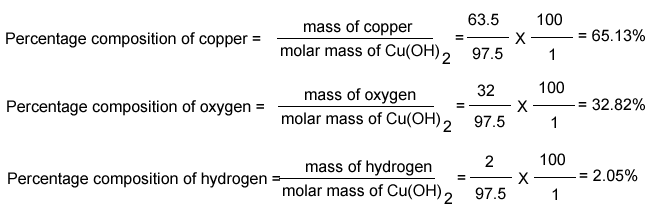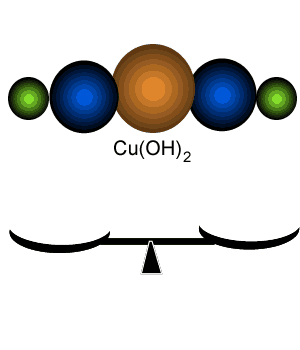Percentage composition of Cu(OH)2 Example Calculate the percentage by mass of each element in Cu(OH)2.What is the mass of copper that is present in 400 grams of Cu(OH)2 ? Since 65.13% of the mass of Cu(OH)2 is due to copper the mass of copper in 400 grams of Cu(OH)2 can be calculated by the equation below. 400 g X 65.13/100 = 260.52 grams 1) Calculate the percentage composition of aluminium sulphate Al2(SO4)3 2) Calculate the mass of aluminium in 400 grams of Al2(SO4)3 3) Calculate the mass of oxygen in 400 grams of Al2(SO4)3 4) Calculate the percentage composition of copper carbonate CuCO3 5) Calculate the mass of copper in 50 grams of CuCO3 Click to hide solution 1) Calculate the percentage composition of aluminium sulphate Al2(SO4)3 15.8%Al, 28.1%S, 56.1%O 2) Calculate the mass of aluminium in 400 grams of Al2(SO4)3 (15.8/100 ) X 400 = 63.2 g 3) Calculate the mass of oxygen in 400 grams of Al2(SO4)3 (56.1 /100) X 400 =224.4 g 4) Calculate the percentage composition of copper carbonate CuCO3 51.4%Cu, 9.7%C, 38.9%O 5) Calculate the mass of copper in 50 grams of CuCO3 (51.4/100) X 50 = 25.7 g   Solution